# Physics Chapter 1 Part 1

31. Aug 2009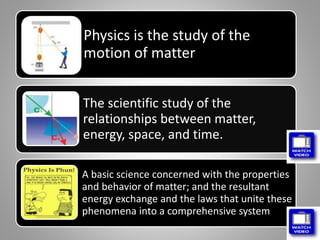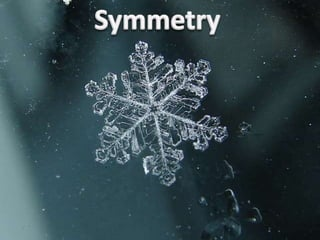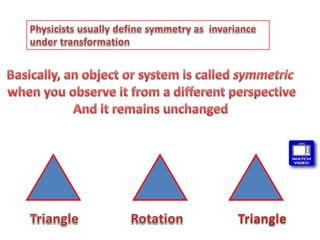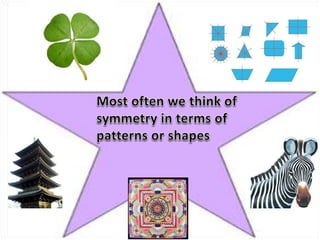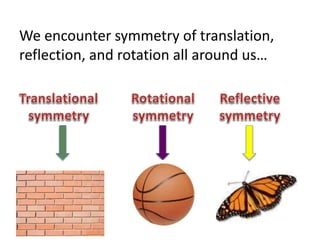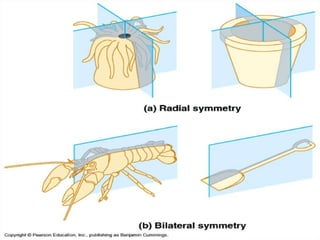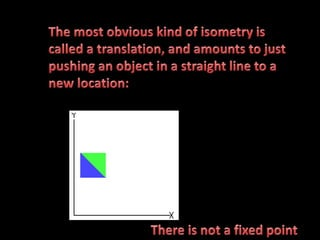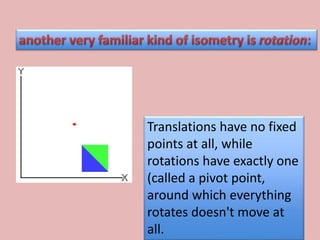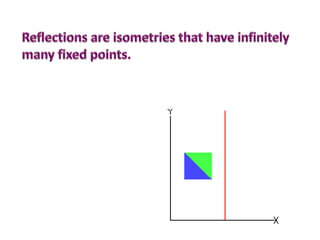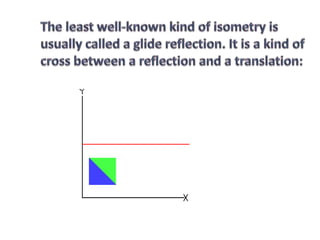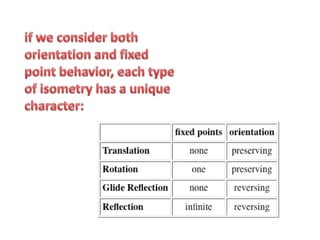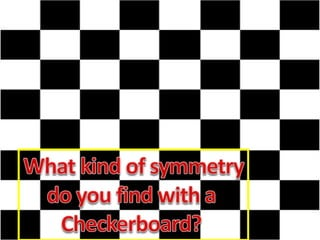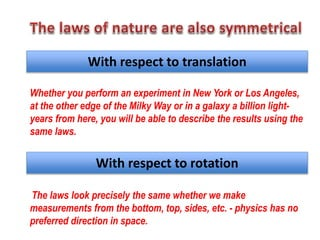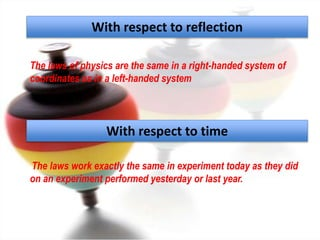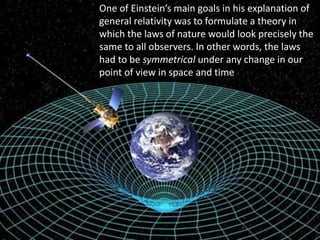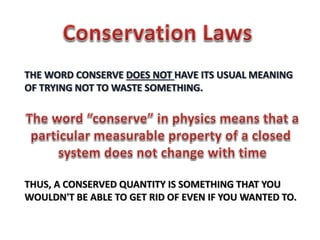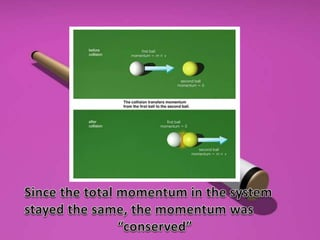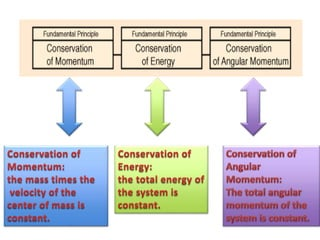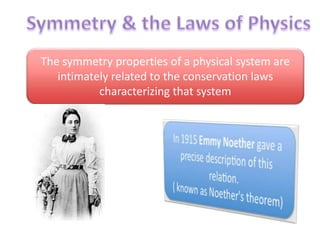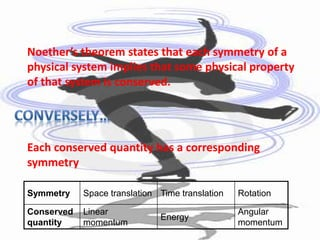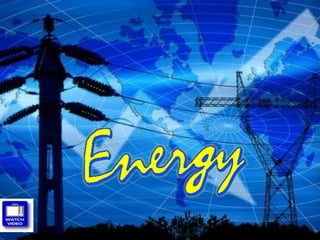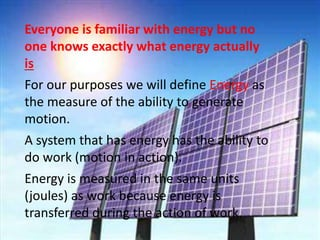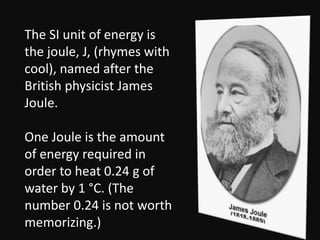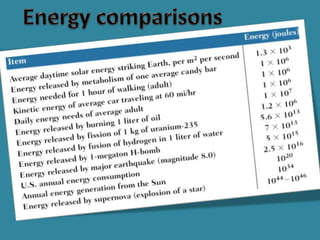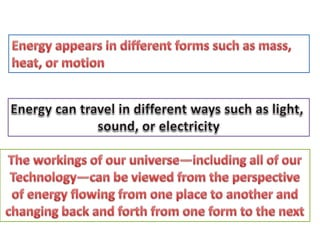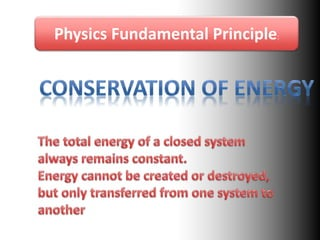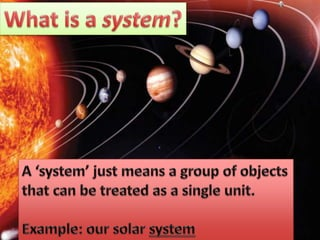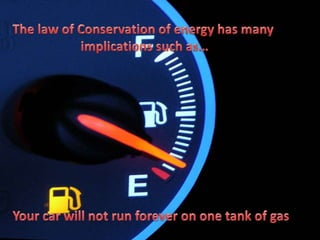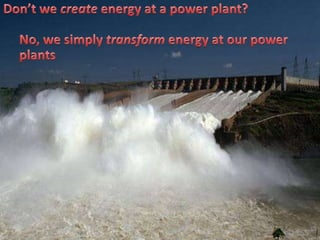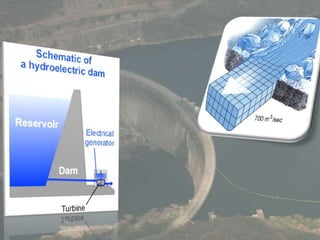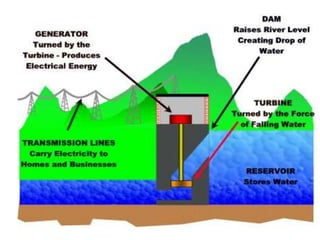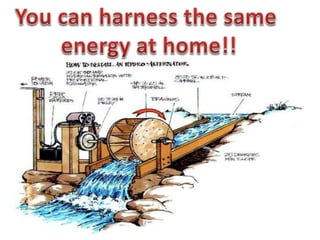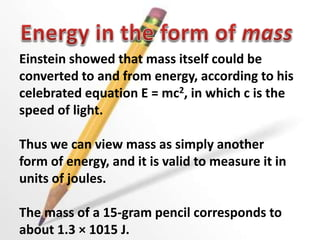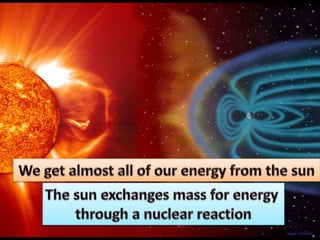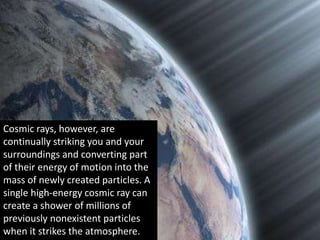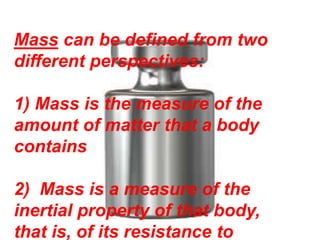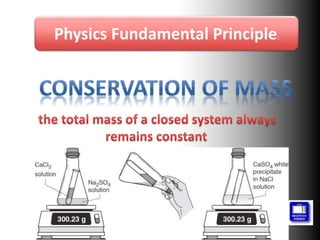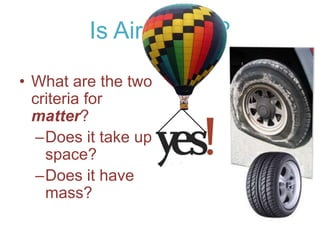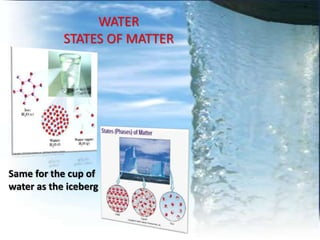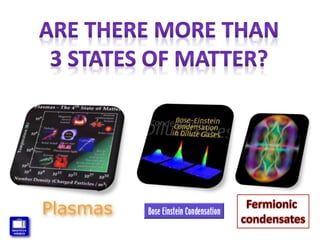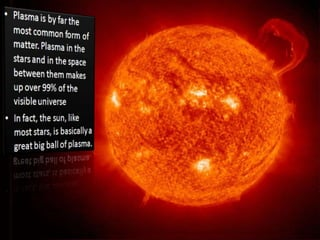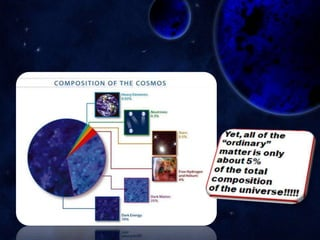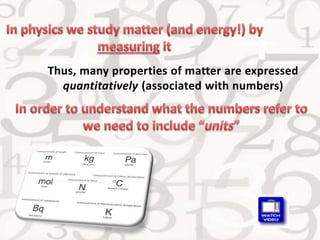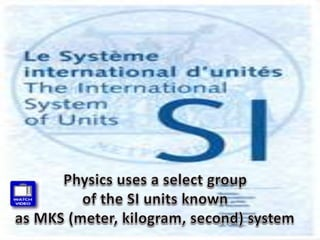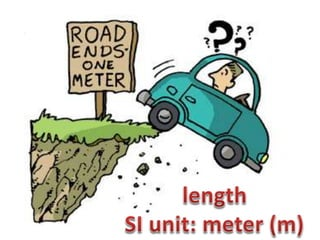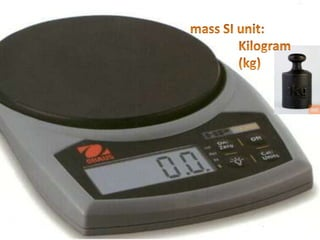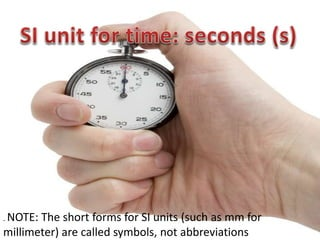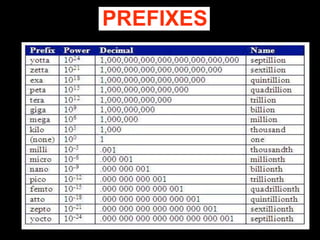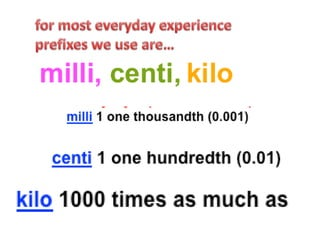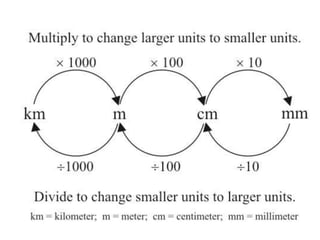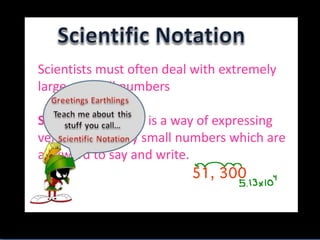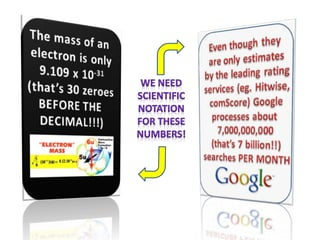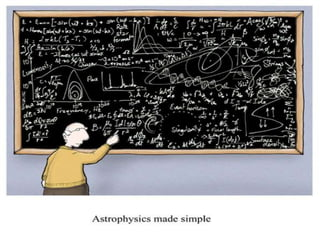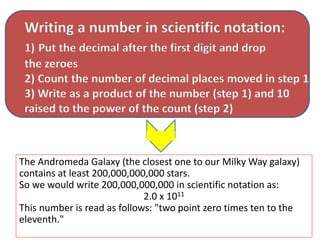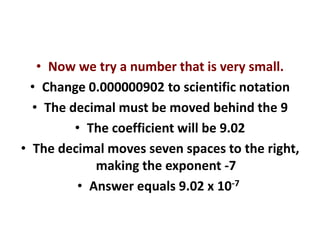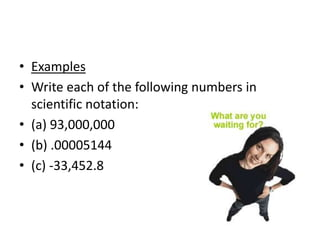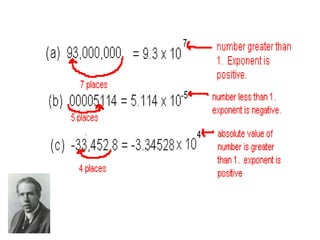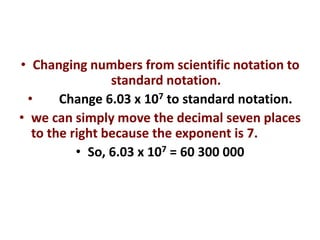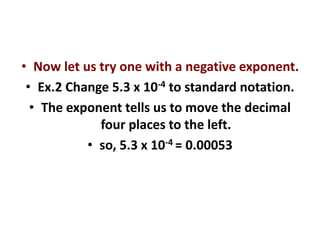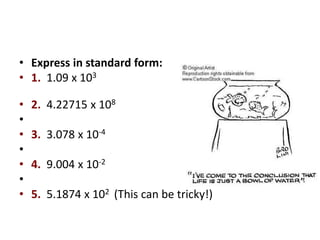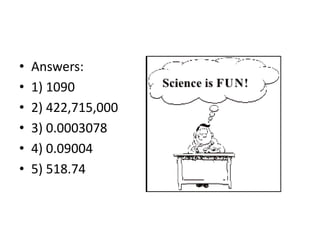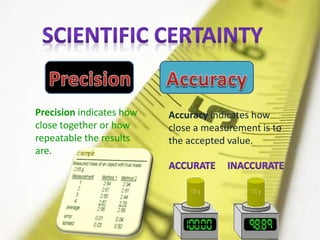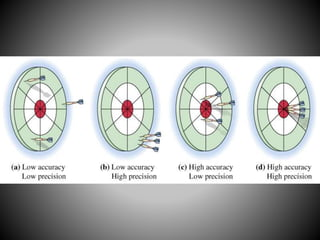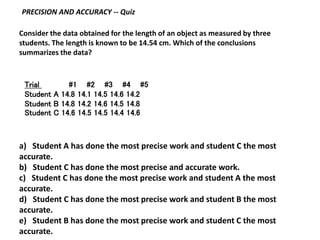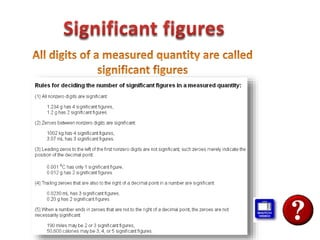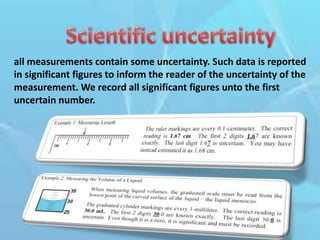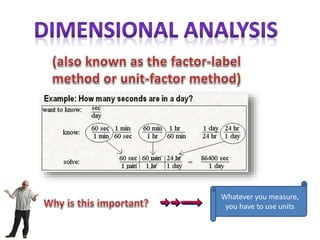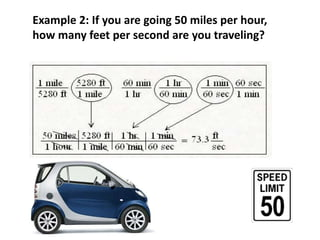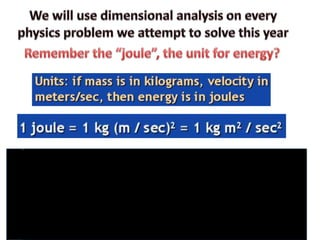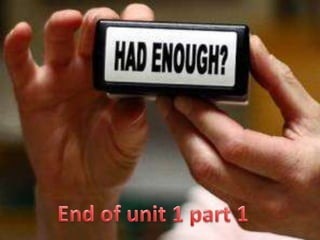1 von 75

### Physics Chapter 1 Part 1

• 2. Physics is the study of the motion of matter The scientific study of the relationships between matter, energy, space, and time. A basic science concerned with the properties and behavior of matter; and the resultant energy exchange and the laws that unite these phenomena into a comprehensive system
• 6. We encounter symmetry of translation, reflection, and rotation all around us…
• 9. Translations have no fixed points at all, while rotations have exactly one (called a pivot point, around which everything rotates doesn't move at all.
• 15. With respect to translation Whether you perform an experiment in New York or Los Angeles, at the other edge of the Milky Way or in a galaxy a billion light- years from here, you will be able to describe the results using the same laws. With respect to rotation The laws look precisely the same whether we make measurements from the bottom, top, sides, etc. - physics has no preferred direction in space.
• 16. With respect to reflection The laws of physics are the same in a right-handed system of coordinates as in a left-handed system With respect to time The laws work exactly the same in experiment today as they did on an experiment performed yesterday or last year.
• 17. One of Einstein’s main goals in his explanation of general relativity was to formulate a theory in which the laws of nature would look precisely the same to all observers. In other words, the laws had to be symmetrical under any change in our point of view in space and time
• 18. THUS, A CONSERVED QUANTITY IS SOMETHING THAT YOU WOULDN'T BE ABLE TO GET RID OF EVEN IF YOU WANTED TO.
• 21. The symmetry properties of a physical system are intimately related to the conservation laws characterizing that system
• 22. Noether’s theorem states that each symmetry of a physical system implies that some physical property of that system is conserved. Each conserved quantity has a corresponding symmetry Symmetry Space translation Time translation Rotation Conserved quantity Linear momentum Energy Angular momentum
• 24. Everyone is familiar with energy but no one knows exactly what energy actually is For our purposes we will define Energy as the measure of the ability to generate motion. A system that has energy has the ability to do work (motion in action). Energy is measured in the same units (joules) as work because energy is transferred during the action of work.
• 25. The SI unit of energy is the joule, J, (rhymes with cool), named after the British physicist James Joule. One Joule is the amount of energy required in order to heat 0.24 g of water by 1 °C. (The number 0.24 is not worth memorizing.)
• 28. Physics Fundamental Principle:
• 35. Einstein showed that mass itself could be converted to and from energy, according to his celebrated equation E = mc2, in which c is the speed of light. Thus we can view mass as simply another form of energy, and it is valid to measure it in units of joules. The mass of a 15-gram pencil corresponds to about 1.3 × 1015 J.
• 37. Cosmic rays, however, are continually striking you and your surroundings and converting part of their energy of motion into the mass of newly created particles. A single high-energy cosmic ray can create a shower of millions of previously nonexistent particles when it strikes the atmosphere.
• 38. Mass can be defined from two different perspectives: 1) Mass is the measure of the amount of matter that a body contains 2) Mass is a measure of the inertial property of that body, that is, of its resistance to
• 39. Physics Fundamental Principle:
• 42. Is Air Matter? • What are the two criteria for matter? –Does it take up space? –Does it have mass?
• 43. WATER STATES OF MATTER Same for the cup of water as the iceberg
• 47. Thus, many properties of matter are expressed quantitatively (associated with numbers)
• 52. . NOTE: The short forms for SI units (such as mm for millimeter) are called symbols, not abbreviations
• 56. Scientists must often deal with extremely large or small numbers Scientific notation is a way of expressing very large or very small numbers which are awkward to say and write.
• 59. Writing a number in scientific notation: 1) Put the decimal after the first digit and drop the zeroes 2) Count the number of decimal places moved in step 1 3) Write as a product of the number (step 1) and 10 raised to the power of the count (step 2) The Andromeda Galaxy (the closest one to our Milky Way galaxy) contains at least 200,000,000,000 stars. So we would write 200,000,000,000 in scientific notation as: 2.0 x 1011 This number is read as follows: "two point zero times ten to the eleventh."
• 60. • Now we try a number that is very small. • Change 0.000000902 to scientific notation • The decimal must be moved behind the 9 • The coefficient will be 9.02 • The decimal moves seven spaces to the right, making the exponent -7 • Answer equals 9.02 x 10-7
• 61. • Examples • Write each of the following numbers in scientific notation: • (a) 93,000,000 • (b) .00005144 • (c) -33,452.8
• 63. • Changing numbers from scientific notation to standard notation. • Change 6.03 x 107 to standard notation. • we can simply move the decimal seven places to the right because the exponent is 7. • So, 6.03 x 107 = 60 300 000
• 64. • Now let us try one with a negative exponent. • Ex.2 Change 5.3 x 10-4 to standard notation. • The exponent tells us to move the decimal four places to the left. • so, 5.3 x 10-4 = 0.00053
• 65. • Express in standard form: • 1. 1.09 x 103 • 2. 4.22715 x 108 • • 3. 3.078 x 10-4 • • 4. 9.004 x 10-2 • • 5. 5.1874 x 102 (This can be tricky!)
• 66. • Answers: • 1) 1090 • 2) 422,715,000 • 3) 0.0003078 • 4) 0.09004 • 5) 518.74
• 67. Accuracy indicates how close a measurement is to the accepted value. Precision indicates how close together or how repeatable the results are.
• 69. Trial #1 #2 #3 #4 #5 Student A 14.8 14.1 14.5 14.6 14.2 Student B 14.8 14.2 14.6 14.5 14.8 Student C 14.6 14.5 14.5 14.4 14.6 PRECISION AND ACCURACY -- Quiz Consider the data obtained for the length of an object as measured by three students. The length is known to be 14.54 cm. Which of the conclusions summarizes the data? a) Student A has done the most precise work and student C the most accurate. b) Student C has done the most precise and accurate work. c) Student C has done the most precise work and student A the most accurate. d) Student C has done the most precise work and student B the most accurate. e) Student B has done the most precise work and student C the most accurate.
• 71. all measurements contain some uncertainty. Such data is reported in significant figures to inform the reader of the uncertainty of the measurement. We record all significant figures unto the first uncertain number.
• 72. Whatever you measure, you have to use units
• 73. Example 2: If you are going 50 miles per hour, how many feet per second are you traveling?